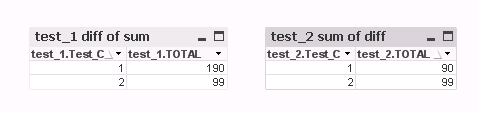# QlikView App Development

Discussion Board for collaboration related to QlikView App Development.

Announcements
Make your voice heard! Participate in the 2020 Wisdom of Crowds® Survey. BEGIN SURVEYNot applicable

## Difference between two expressions

test:

[

Test_A, Test_B, Test_C

10.001, 20, 1

31, 1, 2

];

QUALIFY * ;

test_1:

load sum(Test_A) - sum(Test_B) as TOTAL

Resident test

group by Test_C;

test_2:

load sum(Test_A - Test_B) as TOTAL

Resident test

group by Test_C;

Is there a difference in this two differents ways to calculate sum ?

(In my example this is exactly the same, but in my application with huges datas there are difference and I think is due to this calculation)

Thanks

Tags (2)
1 Solution

Accepted Solutions
Highlighted
Honored Contributor II

## Re: Difference between two expressions

Hi.

In some cases they can give different results.

Suppose that for some records the Test_B is null(). Then the second expression  will skip the whole record even if the Test_A is not null, because Test_A - null() = null()

3 Replies
Highlighted
Honored Contributor II

## Re: Difference between two expressions

Hi.

In some cases they can give different results.

Suppose that for some records the Test_B is null(). Then the second expression  will skip the whole record even if the Test_A is not null, because Test_A - null() = null()

HighlightedMVP

## Re: Difference between two expressions

maybe if you have some null in your application data

resultscript

test:

load rowno() as id, Test_A, if(Test_B='-',null(),Test_B) as Test_B, Test_C;

[

Test_A, Test_B, Test_C

100, 10, 1

100, 1, 2

100,-,1

];

QUALIFY * ;

test_1:

load Test_C, sum(Test_A) - sum(Test_B) as TOTAL

Resident test

group by Test_C;

test_2:

load Test_C, sum(Test_A - Test_B) as TOTAL

Resident test

group by Test_C;

Highlighted
Esteemed Contributor

## Re: Difference between two expressions

first expression is f sum of differnce

where as second is difference of sum